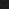JavaPerspective.com  >   Beginner Tutorials  >   5. Java in Practice  >   5.8. Java code exercises  >   5.8.7. Code exercise 7

5.8.7. Code exercise 7
Last updated: 27 January 2013.

Write a method named addCharactersLeft(String str, char c, int desiredLength) that takes 3 arguments. If the length of the string argument str is less than the integer argument desiredLength, then the character argument c is added to the left of str in order to get a string whose length equals desiredLength. The method then returns the obtained string. If the length of the string argument str is greater than the integer argument desiredLength, then str is returned.

For example, if str is "33", c is '0' and desiredLength is 5, the returned string is 00033.

If str is "33", c is '0' and desiredLength is 1, the returned string is 33.

If str is null, null is returned. If desiredLength is negative, null is returned.

public final class Practice {

public String addCharactersLeft(String str, char c, int desiredLength){

if(str == null)

return null;

if(desiredLength < 0)

return null;

if(str.length() < desiredLength){

StringBuffer stringBuffer = new StringBuffer(str);

while(stringBuffer.length() < desiredLength)

stringBuffer.insert(0, c);

return stringBuffer.toString();

}

return str;

}

}

You are here :  JavaPerspective.com  >   Beginner Tutorials  >   5. Java in Practice  >   5.8. Java code exercises  >   5.8.7. Code exercise 7
Next tutorial :  JavaPerspective.com  >   Beginner Tutorials  >   5. Java in Practice  >   5.8. Java code exercises  >   5.8.8. Code exercise 8